# Jacobi inversion problem

(diff) ← Older revision | Latest revision (diff) | Newer revision → (diff)

The problem of inverting the Abelian integrals (cf. Abelian integral) of the first kind of an arbitrary algebraic function field (cf. Algebraic function). In other words, the problem of inverting the Abelian integrals of the first kind on the compact Riemann surfaceof genusthat corresponds to a given algebraic equation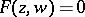.

Letbe a basis of the Abelian differentials of the first kind on(cf. Abelian differential). The inversion of a single Abelian integral, for example,, that is, the representation of all possible rational functions of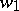, in particular, the representation ofas a function of,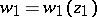, is only meaningful when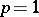. In this case one speaks of the inversion of an elliptic integral, and this leads to doubly-periodic elliptic functions (cf. Elliptic function). For example, the inversion of an integral of the first kind in Legendre normal form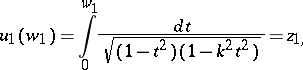leads to the Jacobi elliptic function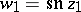(cf. Jacobi elliptic functions).

As C.G.J. Jacobi already observed (1832), the inversion problem for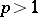must be considered for the set of all Abelian integrals of the first kind,, since one has to obtain functions with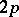periods. In the general case when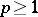, the rational statement of the Jacobi inversion problem is as follows: Suppose one is given a system of equalities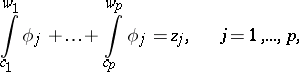(1)

where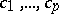are fixed points on,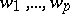are variable points onandare arbitrary but given complex numbers. It is required to find how and under what conditions the system (1) can be inverted, that is, to obtain a representation of all possible symmetric rational functions of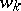,, as functions of.

Since the Abelian integrals in (1), as functions of the upper limit, depend on the form of the path onthat joins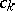and, they are multiple-valued: When the path is varied they can increase by an integer linear combination of periods. Hence it follows that (1) is essentially a system of congruences modulo the periods of the differentials. The values of the functions of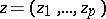obtained by solving the Jacobi inversion problem must remain unchanged when the argument is increased by any integer combination of the periods of the differentials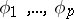. Consequently, they are special Abelian functions withindependent periods (cf. Abelian function).

For the case, that is, for an elliptic integral, the elliptic functions solving the inversion problem can be constructed by using the comparatively-simple Jacobi theta-functions in a single complex variable, and meromorphic elliptic functions can be constructed as quotients of entire theta-functions. It is also possible to solve the general Jacobi inversion problem by using the theta-functions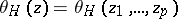of the first order incomplex variables with half-integer characteristics(cf. Theta-function).

The period matrixof the basis Abelian differentials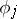has the form(2)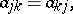and the Riemann relations (see Abelian function) between the periods ensure that the series representing the theta-functions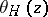, constructed in terms of, converge uniformly on compact sets in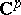. By using the theta-function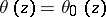of zero characteristic one can construct the superposition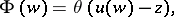where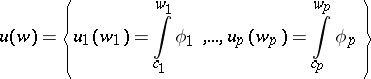is the vector of Abelian integrals and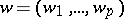is a system of points of;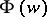is called the Riemann theta-function. For a given set of numbers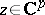, eitherhas ona unique system of zeros(the normal case), or it vanishes identically (the exceptional case). The zeros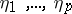give a solution to the Jacobi inversion problem. The exceptional pointsfor which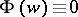form ina set of lower dimension.

Explicit expressions for special Abelian functions that completely solve the Jacobi inversion problem can be constructed by using quotients of theta-functions of the form, where the common denominator is the theta-function of zero characteristic. When periods are added to the argument, the theta-functions are multiplied by specific multipliers. For quotients of theta-functions, after cancellation the only non-trivial multiplier is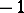. Consequently, the squares of the quotients are unchanged when periods are added to the argument, and one obtains Abelian functions withperiods.

Closely related to the Jacobi inversion problem is the important problem of constructing for a given system of theta-functionswith a common matrixsatisfying the convergence conditions the corresponding algebraic function fields and the corresponding Riemann surface. For this construction to be possible, the distinct elements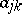of(there are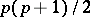of them) must satisfy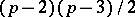additional relations, the investigation of which whenis a very difficult problem (see , , , ).

How to Cite This Entry:
Jacobi inversion problem. Encyclopedia of Mathematics. URL: http://encyclopediaofmath.org/index.php?title=Jacobi_inversion_problem&oldid=11287
This article was adapted from an original article by E.D. Solomentsev (originator), which appeared in Encyclopedia of Mathematics - ISBN 1402006098. See original article